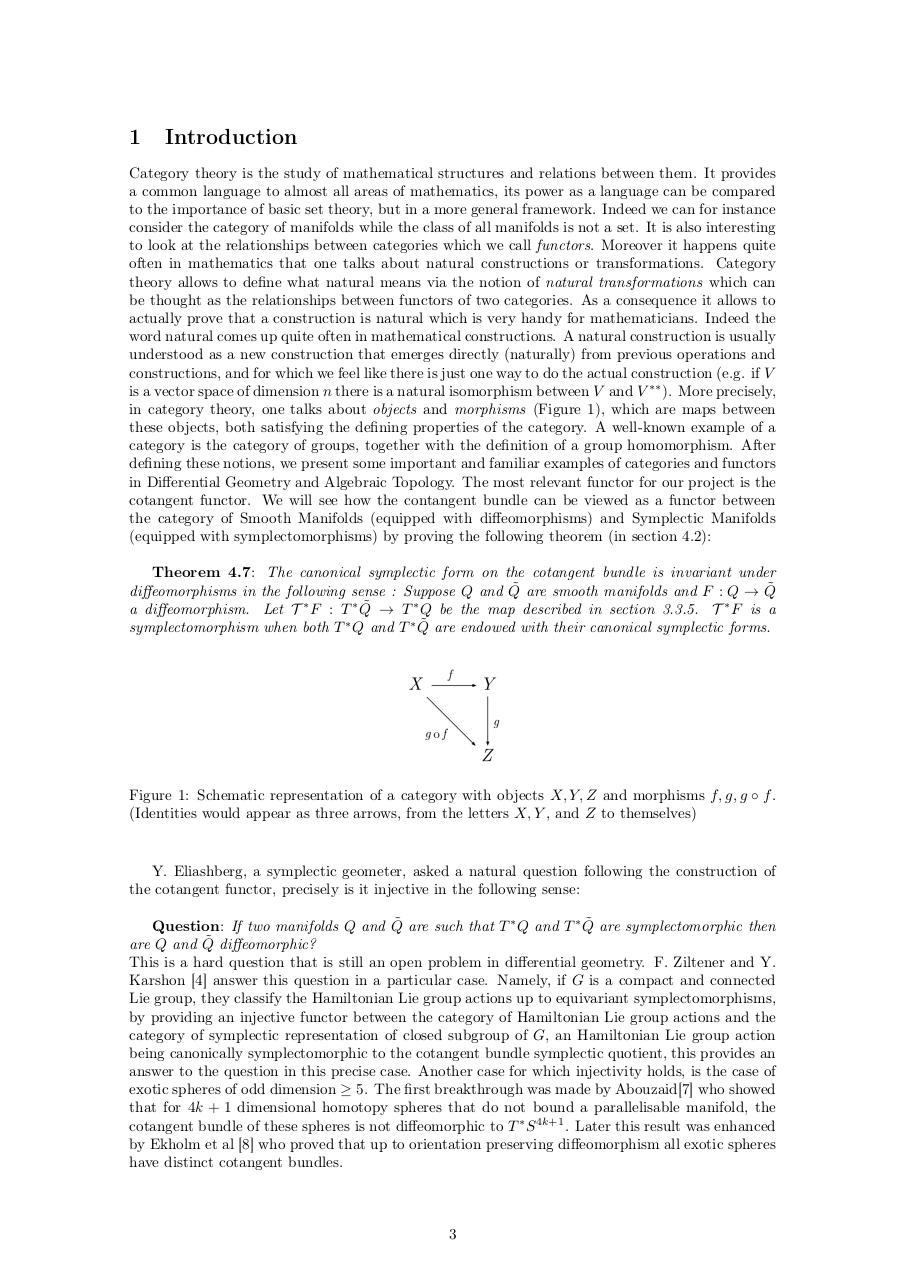# Functors in Differential Geometry.pdfPage 1 2 34514

#### Text preview

1

Introduction

Category theory is the study of mathematical structures and relations between them. It provides
a common language to almost all areas of mathematics, its power as a language can be compared
to the importance of basic set theory, but in a more general framework. Indeed we can for instance
consider the category of manifolds while the class of all manifolds is not a set. It is also interesting
to look at the relationships between categories which we call functors. Moreover it happens quite
often in mathematics that one talks about natural constructions or transformations. Category
theory allows to define what natural means via the notion of natural transformations which can
be thought as the relationships between functors of two categories. As a consequence it allows to
actually prove that a construction is natural which is very handy for mathematicians. Indeed the
word natural comes up quite often in mathematical constructions. A natural construction is usually
understood as a new construction that emerges directly (naturally) from previous operations and
constructions, and for which we feel like there is just one way to do the actual construction (e.g. if V
is a vector space of dimension n there is a natural isomorphism between V and V ∗∗ ). More precisely,
in category theory, one talks about objects and morphisms (Figure 1), which are maps between
these objects, both satisfying the defining properties of the category. A well-known example of a
category is the category of groups, together with the definition of a group homomorphism. After
defining these notions, we present some important and familiar examples of categories and functors
in Differential Geometry and Algebraic Topology. The most relevant functor for our project is the
cotangent functor. We will see how the contangent bundle can be viewed as a functor between
the category of Smooth Manifolds (equipped with diffeomorphisms) and Symplectic Manifolds
(equipped with symplectomorphisms) by proving the following theorem (in section 4.2):
Theorem 4.7: The canonical symplectic form on the cotangent bundle is invariant under
˜ are smooth manifolds and F : Q → Q
˜
diffeomorphisms in the following sense : Suppose Q and Q
˜ → T ∗ Q be the map described in section 3.3.5. T ∗ F is a
a diffeomorphism. Let T ∗ F : T ∗ Q
˜ are endowed with their canonical symplectic forms.
symplectomorphism when both T ∗ Q and T ∗ Q

Figure 1: Schematic representation of a category with objects X, Y, Z and morphisms f, g, g ◦ f .
(Identities would appear as three arrows, from the letters X, Y , and Z to themselves)

Y. Eliashberg, a symplectic geometer, asked a natural question following the construction of
the cotangent functor, precisely is it injective in the following sense:
˜ are such that T ∗ Q and T ∗ Q
˜ are symplectomorphic then
Question: If two manifolds Q and Q
˜
are Q and Q diffeomorphic?
This is a hard question that is still an open problem in differential geometry. F. Ziltener and Y.
Karshon  answer this question in a particular case. Namely, if G is a compact and connected
Lie group, they classify the Hamiltonian Lie group actions up to equivariant symplectomorphisms,
by providing an injective functor between the category of Hamiltonian Lie group actions and the
category of symplectic representation of closed subgroup of G, an Hamiltonian Lie group action
being canonically symplectomorphic to the cotangent bundle symplectic quotient, this provides an
answer to the question in this precise case. Another case for which injectivity holds, is the case of
exotic spheres of odd dimension ≥ 5. The first breakthrough was made by Abouzaid who showed
that for 4k + 1 dimensional homotopy spheres that do not bound a parallelisable manifold, the
cotangent bundle of these spheres is not diffeomorphic to T ∗ S 4k+1 . Later this result was enhanced
by Ekholm et al  who proved that up to orientation preserving diffeomorphism all exotic spheres
have distinct cotangent bundles.

3